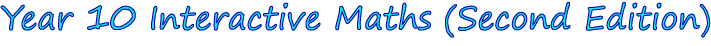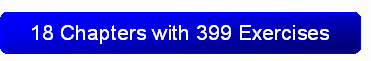1. Algebraic Expressions
2. Linear Equations and Inequalities
3. Linear Graphs
4. Simultaneous Equations
5. Probability
6. Geometry
7. Consumer Arithmetic
8. Indices
9. Surds
10. Factorisation Techniques
11. Rational Expressions
14. Measurement
15. Trigonometry
16. Statistics
17. Variation
18. RevisionThe Year 10 Interactive Maths software is compatible with both Windows® and Mac® computers.  Discover more about Year 10 Interactive Maths by:

• Viewing the 17 chapter screen shots shown below that show step-by-step solutions in G S Rehill's proven writing style.  Students use the solutions after answering questions from the interactive exercises to identify mistakes in their working and to reinforce concepts, reasoning or mathematical laws.
• Clicking Mathematics Software Tutorials to watch 2 tutorials that show how students use the mathematics software.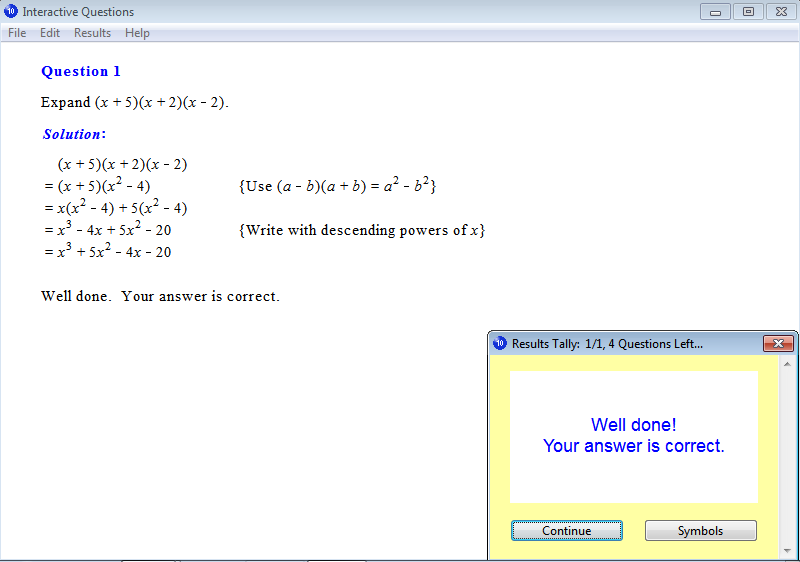From Chapter 1: Algebraic Expressions, Exercise 17: Expanding Three Terms.From Chapter 2: Linear Equations and Inequalities, Exercise 46: Substitution.From Chapter 3: Linear Graphs, Exercise 3: Applications of Gradients.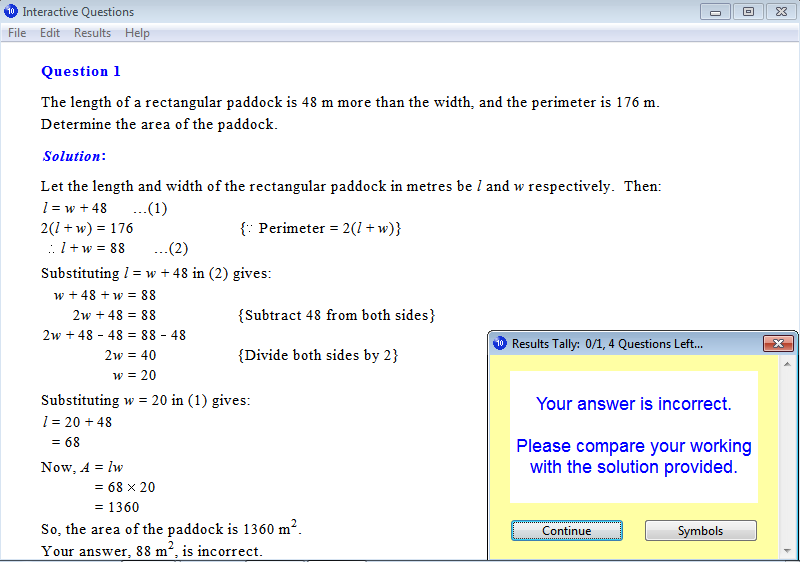From Chapter 4: Simultaneous Equations, Exercise 13: Area Problems.From Chapter 5: Probability, Exercise 14: Addition Law of Probabilities.From Chapter 6: Geometry, Exercise 23: Cyclic Quadrilaterals.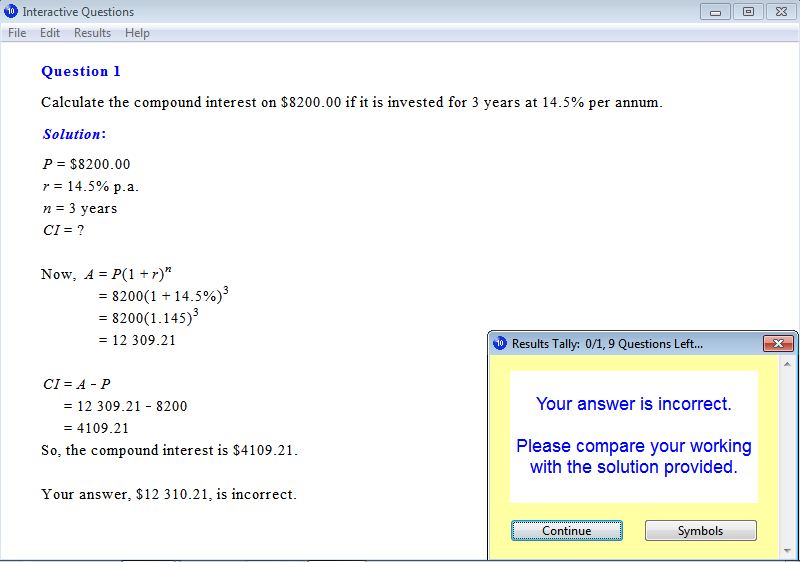From Chapter 7: Consumer Arithmetic, Exercise 22: Compound Interest.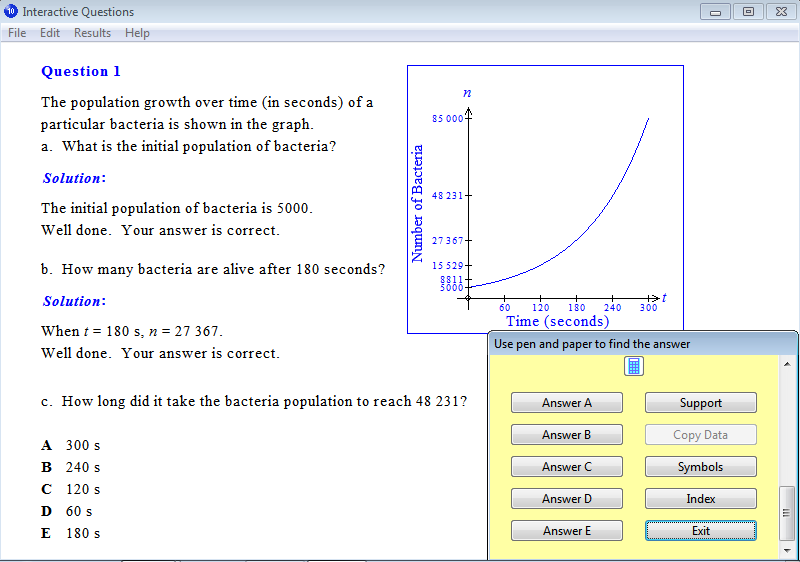From Chapter 8: Indices, Exercise 26: Exponential Graphs.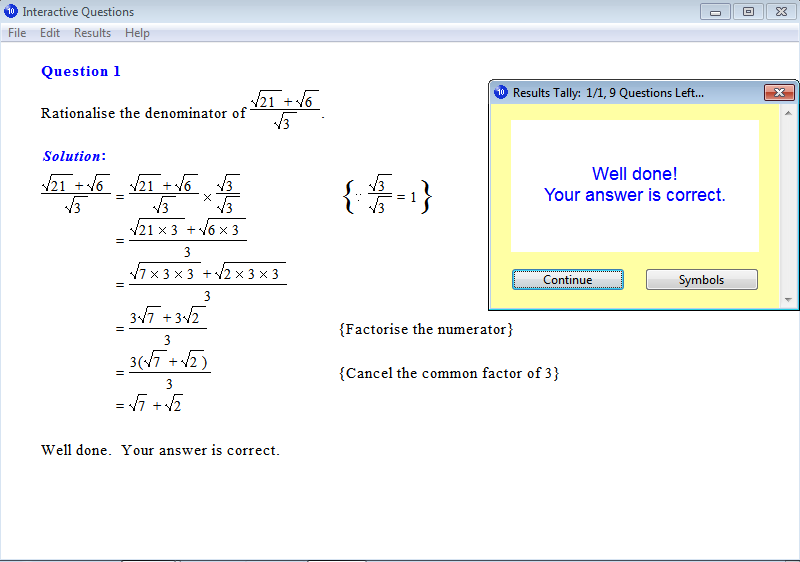From Chapter 9: Surds, Exercise 21: Rationalisation of the Denominator.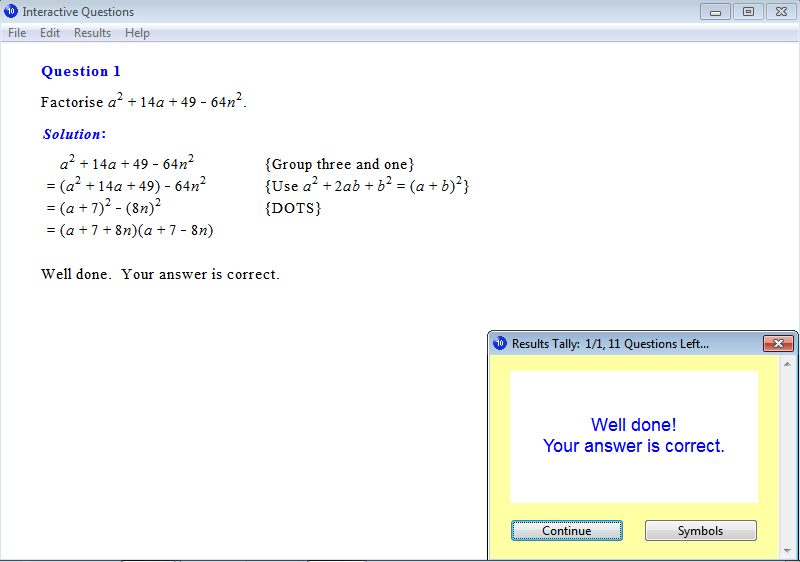From Chapter 10: Factorisation Techniques, Exercise 20: Factorisation by Grouping 'Three and One'.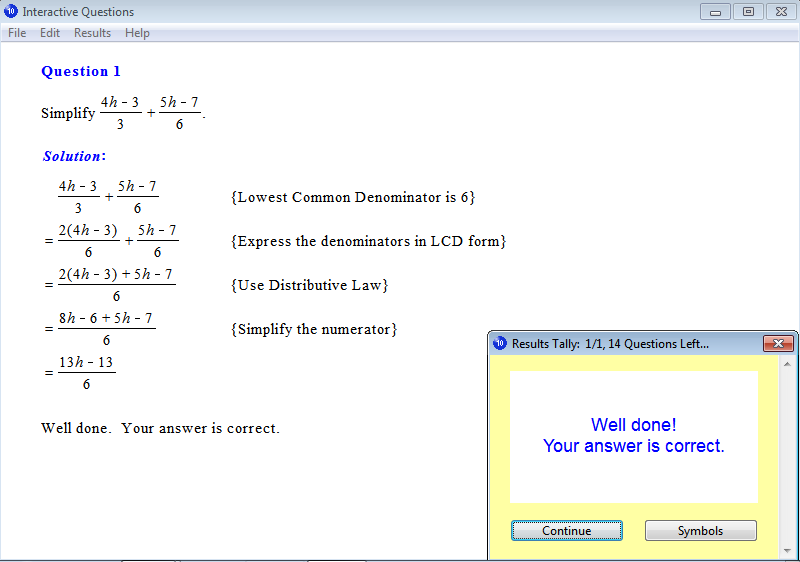From Chapter 11: Rational Expressions, Exercise 22: Addition and Subtraction of Algebraic Expressions.From Chapter 12: Quadratic Equations, Exercise 15: Problem Solving.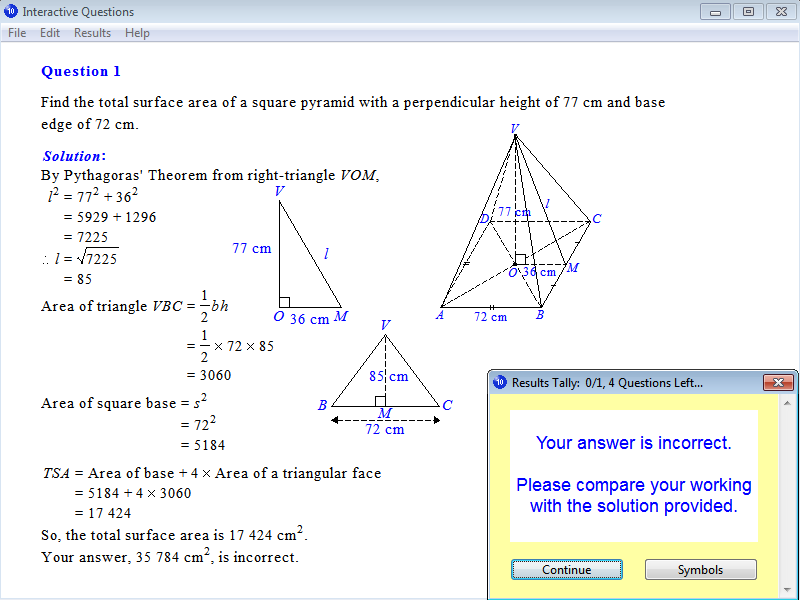From Chapter 14: Measurement, Exercise 24: Total Surface Area of a Pyramid.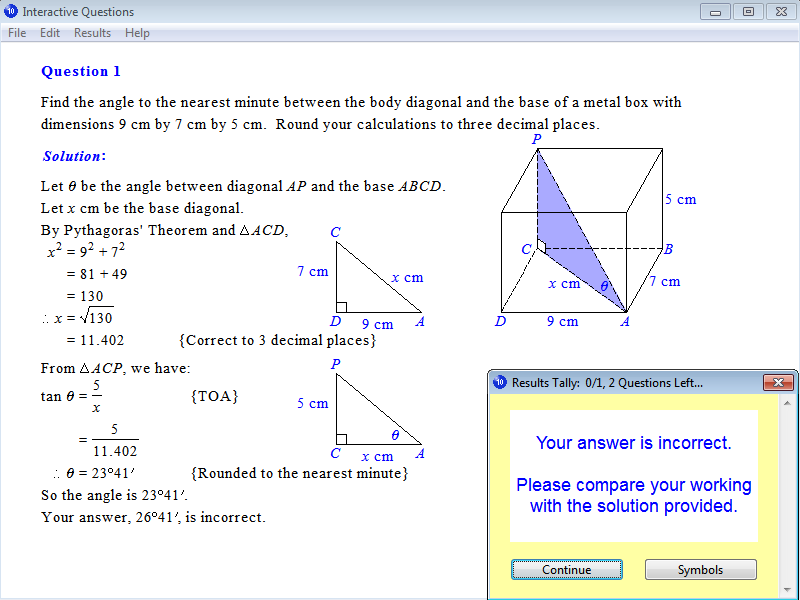From Chapter 15: Trigonometry, Exercise 28: Three-Dimensional Problems.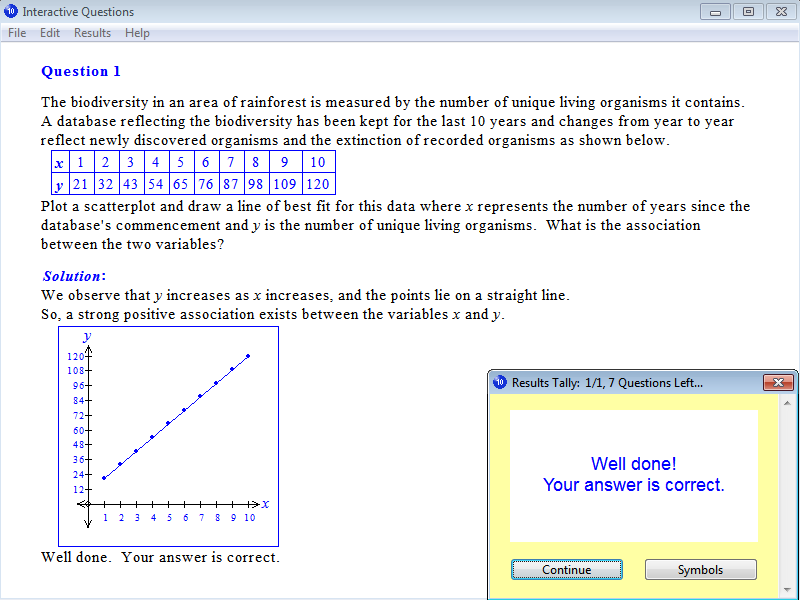From Chapter 16: Statistics, Exercise 11: Line of Best Fit.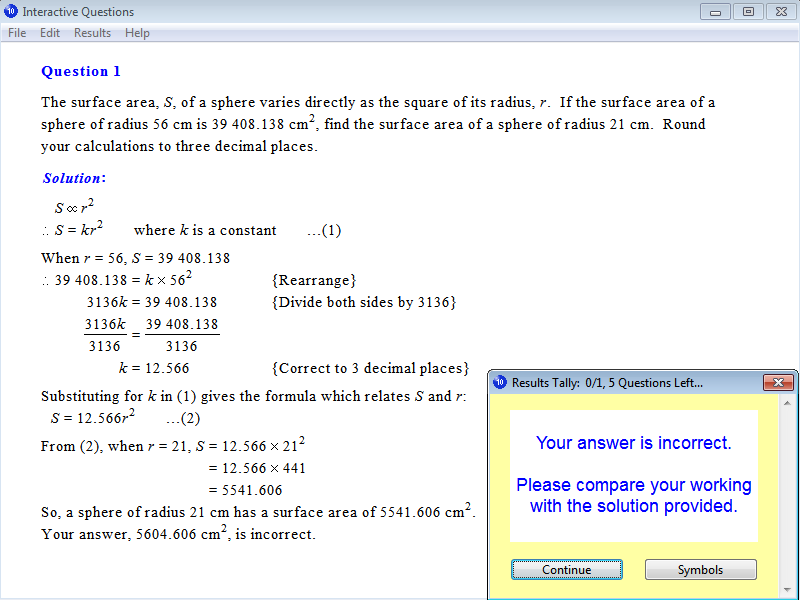From Chapter 17: Variation, Exercise 11: Variation as the Square, Cube or Square Root.

Parents can order a 12-month Homework Licence for \$19.95 (AUD) per student by clicking this order button: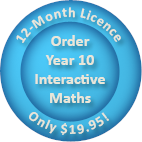Tutors may order Tutor Licences online that feature the interactive exercises as well as worksheets, tests and solution sheets.